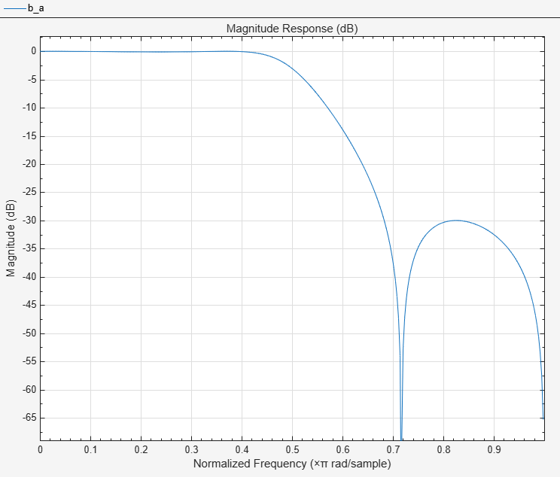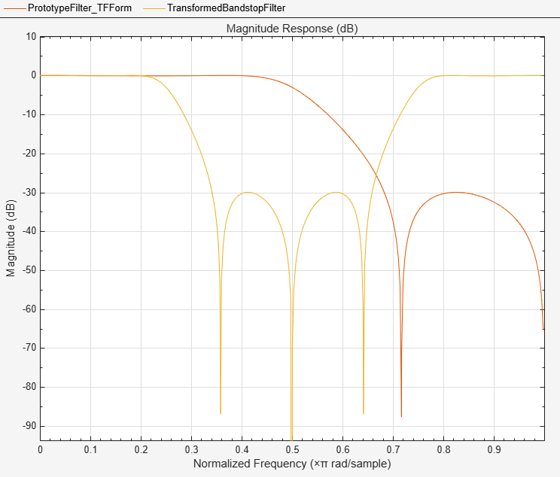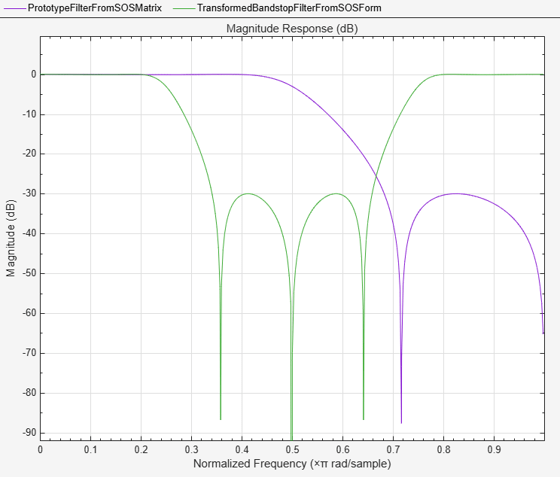# iirlp2bs

Transform IIR lowpass filter to IIR bandstop filter

## Syntax

``````[num,den,allpassNum,allpassDen] = iirlp2bs(b,a,wo,wt)``````

## Description

example

``````[num,den,allpassNum,allpassDen] = iirlp2bs(b,a,wo,wt)``` transforms an IIR lowpass filter to an IIR bandstop filter.The lowpass filter is specified using the numerator and denominator coefficients `b` and `a` respectively. The function returns the numerator and denominator coefficients of the transformed bandstop digital filter. The function also returns the numerator and denominator coefficients of the allpass mapping filter, `allpassNum` and `allpassDen` respectively.For more details on the transformation, see IIR Lowpass Filter to IIR Bandstop Filter Transformation.Frequencies must be normalized to be between 0 and 1, with 1 corresponding to half the sample rate.```

## Examples

collapse all

Transform a lowpass IIR filter to a bandstop filter using the `iirlp2bs` function.

Input Lowpass IIR Filter

Design a prototype real IIR lowpass elliptic filter with a gain of about –3 dB at 0.5π rad/sample.

```[b,a] = ellip(3,0.1,30,0.409); fvtool(b,a)```Transform Filter Using `iirlp2bs`

Transform the prototype lowpass filter into a bandstop filter by placing the cutoff frequencies of the prototype filter at 0.25π and 0.75π.

Specify the prototype filter as a vector of numerator and denominator coefficients, `b` and `a` respectively.

`[num,den] = iirlp2bs(b,a,0.5,[0.25 0.75]);`

Compare the magnitude response of the filters using FVTool.

```hfvt = fvtool(b,a,num,den); legend(hfvt,"Prototype Filter (TF Form)",... "Transformed Bandstop Filter")```Alternatively, you can also specify the input lowpass IIR filter as a matrix of coefficients. Pass the second-order section matrices as inputs. The transformed filter is a fourth-order section filter given by the numerator and the denominator coefficients `num2` and `den2`.

```ss = tf2sos(b,a); [num2,den2] = iirlp2bs(ss(:,1:3),ss(:,4:6),0.5,[0.25 0.75]);```

Compare the magnitude response of the filters using FVTool.

To visualize the magnitude response of the fourth-order section filter, first pass the filter coefficients to the `dsp.FourthOrderSectionFilter` object. Then, use this object as an input to the FVTool.

```fos = dsp.FourthOrderSectionFilter(Numerator=num2,... Denominator=den2); hvft = fvtool(ss,fos); legend(hvft,"Prototype Filter (SOS Form)",... "Transformed Bandstop Filter")```## Input Arguments

collapse all

Numerator coefficients of the prototype lowpass IIR filter, specified as either:

• Row vector –– Specifies the values of [b0, b1, …, bn], given this transfer function form:

`$H\left(z\right)=\frac{B\left(z\right)}{A\left(z\right)}=\frac{{b}_{0}+{b}_{1}{z}^{-1}+\cdots +{b}_{n}{z}^{-n}}{{a}_{0}+{a}_{1}{z}^{-1}+\cdots +{a}_{n}{z}^{-n}},$`

where n is the order of the filter.

• Matrix –– Specifies the numerator coefficients in the form of an P-by-(Q+1) matrix, where P is the number of filter sections and Q is the order of each filter section. If Q = 2, the filter is a second-order section filter. For higher-order sections, make Q > 2.

`$b=\left[\begin{array}{ccccc}{b}_{01}& {b}_{11}& {b}_{21}& ...& {b}_{Q1}\\ {b}_{02}& {b}_{12}& {b}_{22}& ...& {b}_{Q2}\\ ⋮& ⋮& ⋮& \ddots & ⋮\\ {b}_{0P}& {b}_{1P}& {b}_{2P}& \cdots & {b}_{QP}\end{array}\right]$`

In the transfer function form, the numerator coefficient matrix bik of the IIR filter can be represented using the following equation:

`$H\left(z\right)=\prod _{k=1}^{P}{H}_{k}\left(z\right)=\prod _{k=1}^{P}\frac{{b}_{0k}+{b}_{1k}{z}^{-1}+{b}_{2k}{z}^{-2}+\cdots +{b}_{Qk}{z}^{-Q}}{{a}_{0k}+{a}_{1k}{z}^{-1}+{a}_{2k}{z}^{-2}+\cdots +{a}_{Qk}{z}^{-Q}},$`

where,

• a –– Denominator coefficients matrix. For more information on how to specify this matrix, see `a`.

• k –– Row index.

• i –– Column index.

When specified in the matrix form, b and a matrices must have the same number of rows (filter sections) Q.

Data Types: `single` | `double`
Complex Number Support: Yes

Denominator coefficients for a prototype lowpass IIR filter, specified as one of these options:

• Row vector –– Specifies the values of [a0, a1, …, an], given this transfer function form:

`$H\left(z\right)=\frac{B\left(z\right)}{A\left(z\right)}=\frac{{b}_{0}+{b}_{1}{z}^{-1}+\cdots +{b}_{n}{z}^{-n}}{{a}_{0}+{a}_{1}{z}^{-1}+\cdots +{a}_{n}{z}^{-n}},$`

where n is the order of the filter.

• Matrix –– Specifies the denominator coefficients in the form of an P-by-(Q+1) matrix, where P is the number of filter sections and Q is the order of each filter section. If Q = 2, the filter is a second-order section filter. For higher-order sections, make Q > 2.

`$a=\left[\begin{array}{ccccc}{a}_{01}& {a}_{11}& {a}_{21}& \cdots & {a}_{Q1}\\ {a}_{02}& {a}_{12}& {a}_{22}& \cdots & {a}_{Q2}\\ ⋮& ⋮& ⋮& \ddots & ⋮\\ {a}_{0P}& {a}_{1P}& {a}_{2P}& \cdots & {a}_{QP}\end{array}\right]$`

In the transfer function form, the denominator coefficient matrix aik of the IIR filter can be represented using the following equation:

`$H\left(z\right)=\prod _{k=1}^{P}{H}_{k}\left(z\right)=\prod _{k=1}^{P}\frac{{b}_{0k}+{b}_{1k}{z}^{-1}+{b}_{2k}{z}^{-2}+\cdots +{b}_{Qk}{z}^{-Q}}{{a}_{0k}+{a}_{1k}{z}^{-1}+{a}_{2k}{z}^{-2}+\cdots +{a}_{Qk}{z}^{-Q}},$`

where,

• b –– Numerator coefficients matrix. For more information on how to specify this matrix, see `b`.

• k –– Row index.

• i –– Column index.

When specified in the matrix form, a and b matrices must have the same number of rows (filter sections) P.

Data Types: `single` | `double`
Complex Number Support: Yes

Frequency value to transform from the prototype filter, specified as a real scalar. Frequency `wo` must be normalized to be between `0` and `1`, with `1` corresponding to half the sample rate.

Data Types: `single` | `double`

Desired frequency locations in the transformed target filter, specified as a two-element vector. Frequencies in `wt` must be normalized to be between `0` and `1`, with `1` corresponding to half the sample rate.

Data Types: `single` | `double`

## Output Arguments

collapse all

Numerator coefficients of the transformed bandstop filter, returned as one of the following:

• Row vector of length 2n+1, where n is the order of the input filter. The `num` output is a row vector when the input coefficients `b` and `a` are row vectors.

• P-by-(2Q+1) matrix, where P is the number of filter sections and Q is the order of each section of the transformed filter. The `num` output is a matrix when the input coefficients `b` and `a` are matrices.

Data Types: `single` | `double`
Complex Number Support: Yes

Denominator coefficients of the transformed bandstop filter, returned as one of the following:

• Row vector of length 2n+1, where n is the order of the input filter. The `den` output is a row vector when the input coefficients `b` and `a` are row vectors.

• P-by-(2Q+1) matrix, where P is the number of filter sections and Q is the order of each section of the transformed filter. The `den` output is a matrix when the input coefficients `b` and `a` are matrices.

Data Types: `single` | `double`

Numerator of the mapping filter, returned as a row vector.

Data Types: `single` | `double`

Denominator of the mapping filter, returned as a row vector.

Data Types: `single` | `double`

collapse all

### IIR Lowpass Filter to IIR Bandstop Filter Transformation

IIR lowpass filter to IIR bandstop filter transformation effectively places one feature of the original filter, located at frequency −`wo`, at the required target frequency location, `wt1`, and the second feature, originally at `wo`, at the new location, `wt2`. Choice of the feature subject to the lowpass to bandstop transformation is not restricted only to the cutoff frequency of an original lowpass filter. You can choose to transform any feature of the original filter like stopband edge, DC, deep minimum in the stopband, or others. It is assumed that `wt2` is greater than `wt1`. Frequencies must be normalized to be between 0 and 1, with 1 corresponding to half the sample rate.

This transformation implements the "Nyquist Mobility," which means that the DC feature stays at DC, but the Nyquist feature moves to a location dependent on the selection of `wo` and `wt`s.

Relative positions of other features of the original filter change in the target filter. This means that it is possible to select two features of the original filter, F1 and F2, with F1 preceding F2. After the transformation feature F2 will precede F1 in the target filter. In addition, the distance between F1 and F2 will not be the same before and after the transformation.

For more details on the lowpass to bandstop frequency transformation, see Digital Frequency Transformations.

 Nowrouzian, B., and A.G. Constantinides. “Prototype Reference Transfer Function Parameters in the Discrete-Time Frequency Transformations.” In Proceedings of the 33rd Midwest Symposium on Circuits and Systems, 1078–82. Calgary, Alta., Canada: IEEE, 1991. https://doi.org/10.1109/MWSCAS.1990.140912.

 Nowrouzian, B., and L.T. Bruton. “Closed-Form Solutions for Discrete-Time Elliptic Transfer Functions.” In  Proceedings of the 35th Midwest Symposium on Circuits and Systems , 784–87. Washington, DC, USA: IEEE, 1992. https://doi.org/10.1109/MWSCAS.1992.271206.

 Constantinides, A.G. “Design of Bandpass Digital Filters.” Proceedings of the IEEE 57, no. 6 (1969): 1229–31. https://doi.org/10.1109/PROC.1969.7216.

 Constantinides, A.G.“Spectral transformations for digital filters.” Proceedings of the IEEE, vol. 117, no. 8: 1585-1590. August 1970.